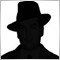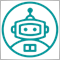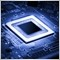• Overview
• Reviews (4)

# Percent b Bollinger BandWidth tfmt5

%b shows you as a percentage where the last price is in relation to the lower and upper Bollinger Bands. If the price is at the moving average line, %b will show on the secondary chart window as a value of 50 which is 50% between the bands. %b can be a negative value (below the lower band) or above 100 (above the upper band) when the price is outside of the bands. This indicator also includes an upper line and lower line that you can specify. By default these are set at 0 and 100 for the 0% and 100% marks for you to easily see the lower and upper bounds of the Bollinger Bands. You can move these lines if you want to see different bounds such as 15 and 85 for when the price crosses the 15% or 85% marks.

Use this together with our free Bollinger BandWidth indicator to effectively use the Bollinger Bands. %b helps you see where the price is in relation to the bands as the volatility expands and contracts. The inputs include:

• BandPeriods: The number of periods used to calculate the moving average in the middle of the Bollinger Bands
• Deviations: The number of standard deviations used to calculate the upper and lower Bollinger Bands
• UpperLevel: The number to add the upper bound line to the indicator
• LowerLevel: The number to add the lower bound line to the indicator

The summarized calculation for %b is

`( last - lower band )  /  ( upper band - lower band )`
Filter:[Deleted] 2019.01.14 07:45

User didn't leave any comment to the rating12010
2018.01.20 20:09

User didn't leave any comment to the rating5709
2017.11.09 14:30

User didn't leave any comment to the rating7209
2016.03.11 04:52

User didn't leave any comment to the rating